voltage is known as the TIME CONSTANT (TC) of the circuit. The charge and discharge curves of a capacitor are shown in figure 3-11. Note that the charge curve is like the curve in figure 3-9, graph (D), and the discharge curve like the curve in figure 3-9, graph (B). ">Custom SearchRC TIME CONSTANT The time required to charge a capacitor to 63 percent (actually 63.2 percent) of full charge or to discharge it to 37 percent (actually 36.8 percent) of its initial voltage is known as the TIME CONSTANT (TC) of the circuit. The charge and discharge curves of a capacitor are shown in figure 3-11. Note that the charge curve is like the curve in figure 3-9, graph (D), and the discharge curve like the curve in figure 3-9, graph (B). Figure 3-11. - RC time constant.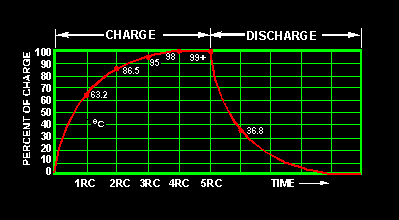The value of the time constant in seconds is equal to the product of the circuit resistance in ohms and the circuit capacitance in farads. The value of one time constant is expressed mathematically as t = RC. Some forms of this formula used in calculating RC time constants are:Q.14 What is the RC time constant of a series RC circuit that contains a 12-megohm resistor and a 12-microfarad capacitor? UNIVERSAL TIME CONSTANT CHART Because the impressed voltage and the values of R and C or R and L in a circuit are usually known, a UNIVERSAL TIME CONSTANT CHART (fig. 3-12) can be used to find the time constant of the circuit. Curve A is a plot of both capacitor voltage during charge and inductor current during growth. Curve B is a plot of both capacitor voltage during discharge and inductor current during decay. Figure 3-12. - Universal time constant chart for RC and RL circuit.The time scale (horizontal scale) is graduated in terms of the RC or L/R time constants so that the curves may be used for any value of R and C or L and R. The voltage and current scales (vertical scales) are graduated in terms of percentage of the maximum voltage or current so that the curves may be used for any value of voltage or current. If the time constant and the initial or final voltage for the circuit in question are known, the voltages across the various parts of the circuit can be obtained from the curves for any time after the switch is closed, either on charge or discharge. The same reasoning is true of the current in the circuit. The following problem illustrates how the universal time constant chart may be used. An RC circuit is to be designed in which a capacitor (C) must charge to 20 percent (0.20) of the maximum charging voltage in 100 microseconds (0.0001 second). Because of other considerations, the resistor (R) must have a value of 20,000 ohms. What value of capacitance is needed?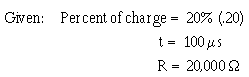Find: The capacitance of capacitor C. Solution: Because the only values given are in units of time and resistance, a variation of the formula to find RC time is used: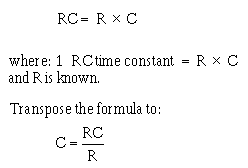Find the value of RC by referring to the universal time constant chart in figure 3-12 and proceed as follows: Locate the 20 point on the vertical scale at the left side of the chart (percentage). Follow the horizontal line from this point to intersect curve A. Follow an imaginary vertical line from the point of intersection on curve A downward to cross the RC scale at the bottom of the chart. Note that the vertical line crosses the horizontal scale at about .22 RC as illustrated below: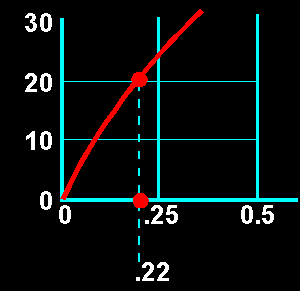The value selected from the graph means that a capacitor (including the one you are solving for) will reach twenty percent of full charge in twenty-two one hundredths (.22) of one RC time constant. Remember that it takes 100 ms for the capacitor to reach 20% of full charge. Since 100 ms is equal to .22 RC (twenty-two one-hundredths), then the time required to reach one RC time constant must be equal to: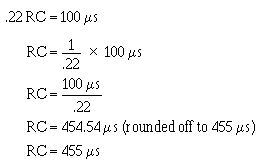Now use the following formula to find C:To summarize the above procedures, the problem and solution are shown below without the step by step explanation.Transpose the RC time constant formula as follows: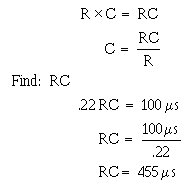Substitute the R and RC values into the formula:The graphs shown in figure 3-11 and 3-12 are not entirely complete. That is, the charge or discharge (or the growth or decay) is not quite complete in 5 RC or 5 L/R time constants. However, when the values reach 0.99 of the maximum (corresponding to 5 RC or 5 L/R), the graphs may be considered accurate enough for all practical purposes. Q.15 A circuit is to be designed in which a capacitor must charge to 40 percent of the maximum charging voltage in 200 microseconds. The resistor to be used has a resistance of 40,000 ohms. What size capacitor must be used? (Use the universal time constant chart in figure 3-12.)Integrated Publishing, Inc. - A (SDVOSB) Service Disabled Veteran Owned Small Business